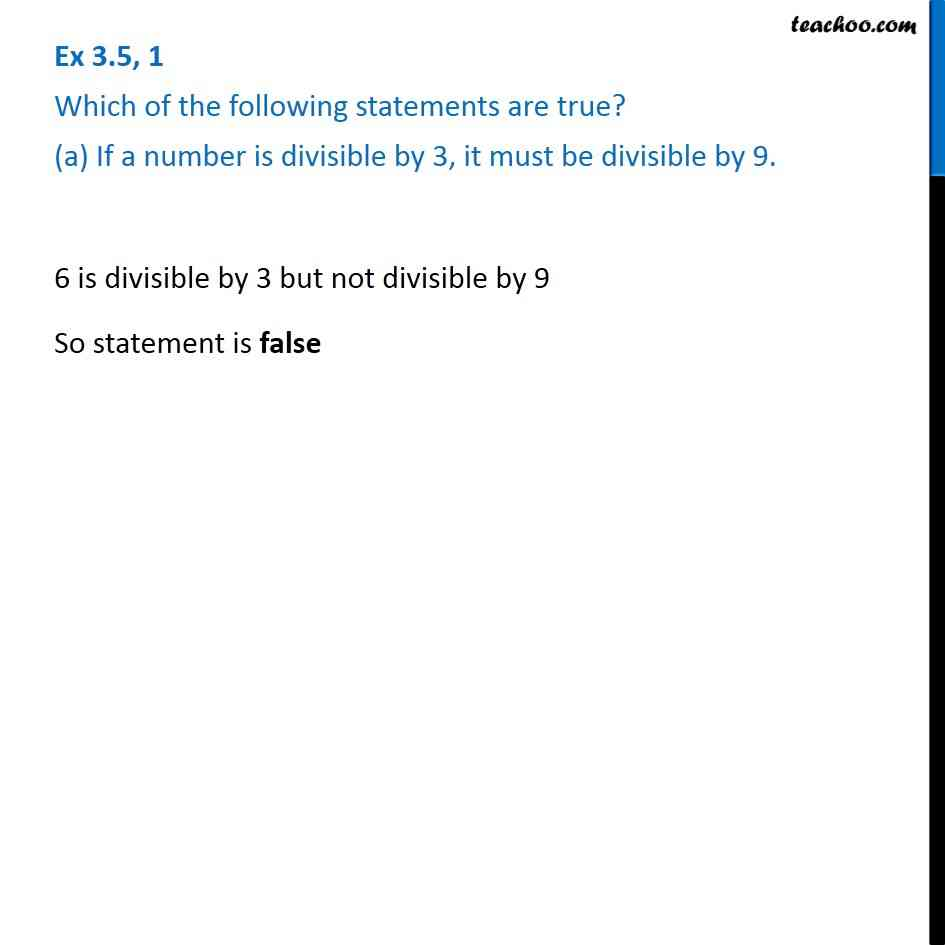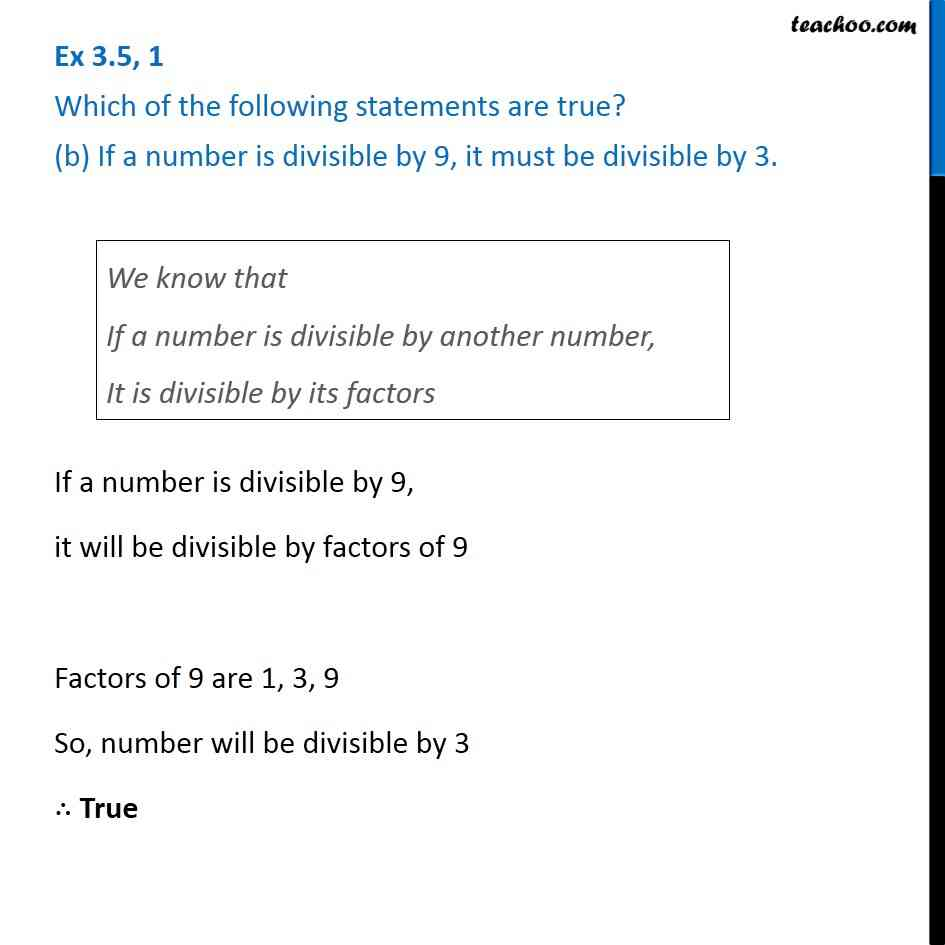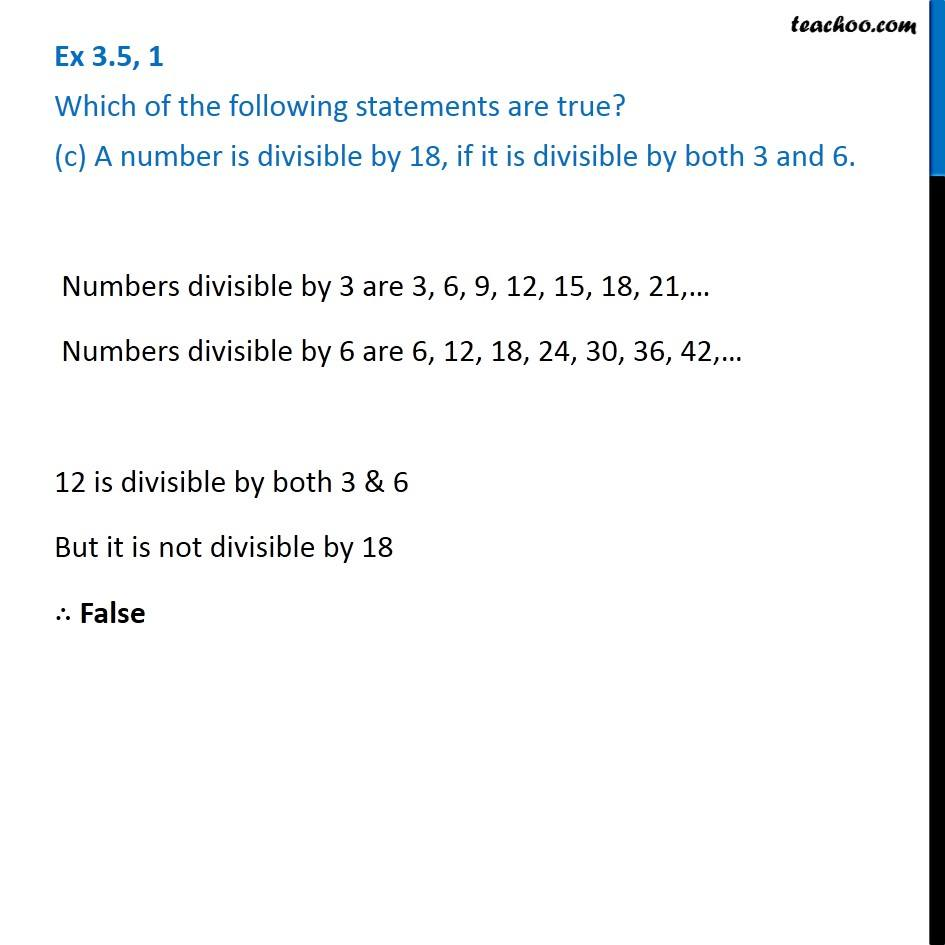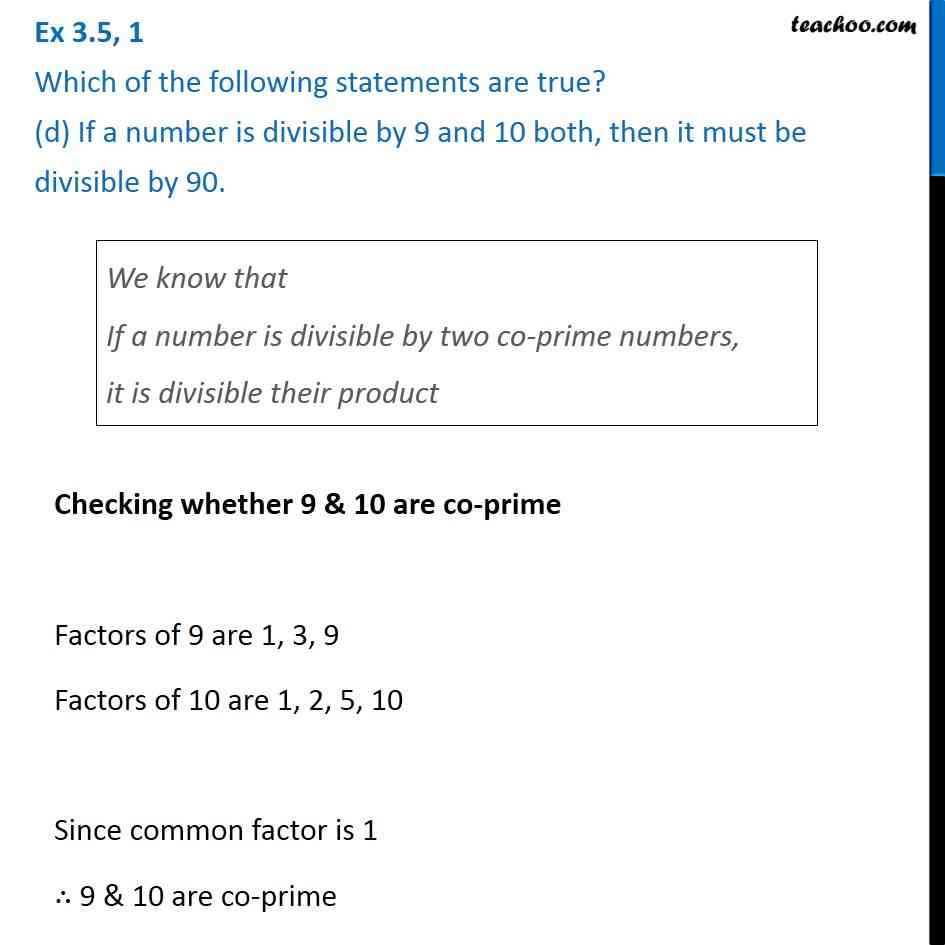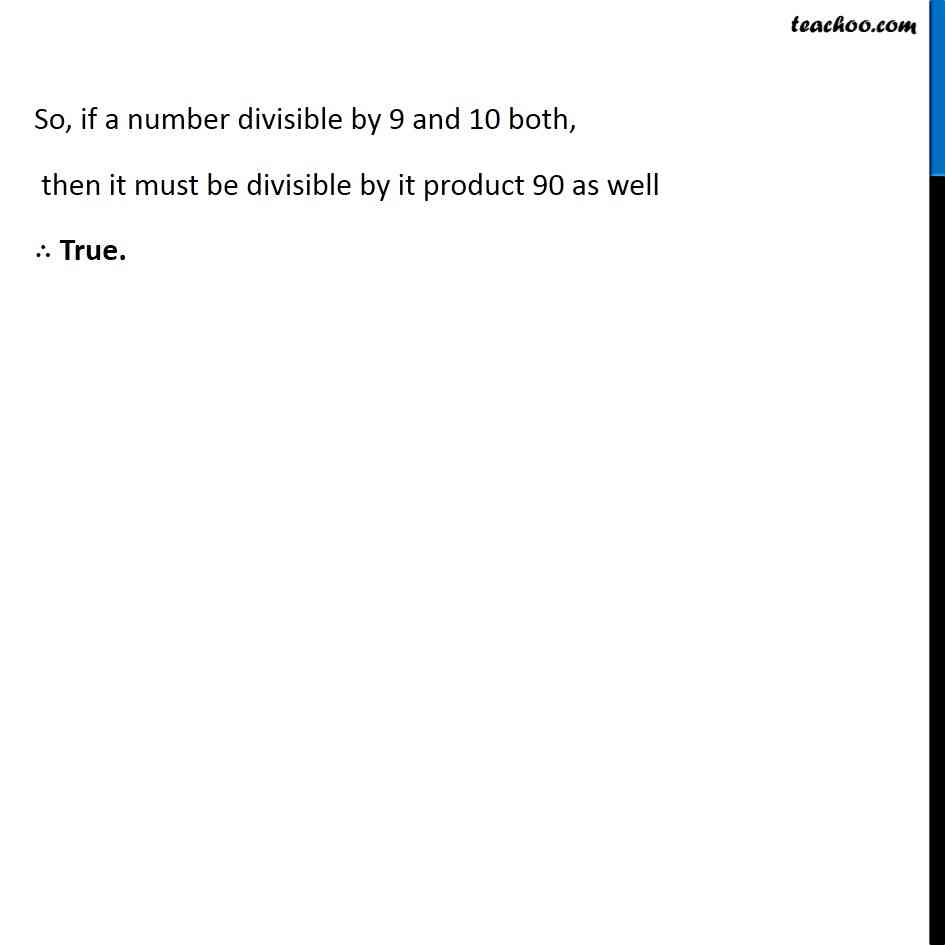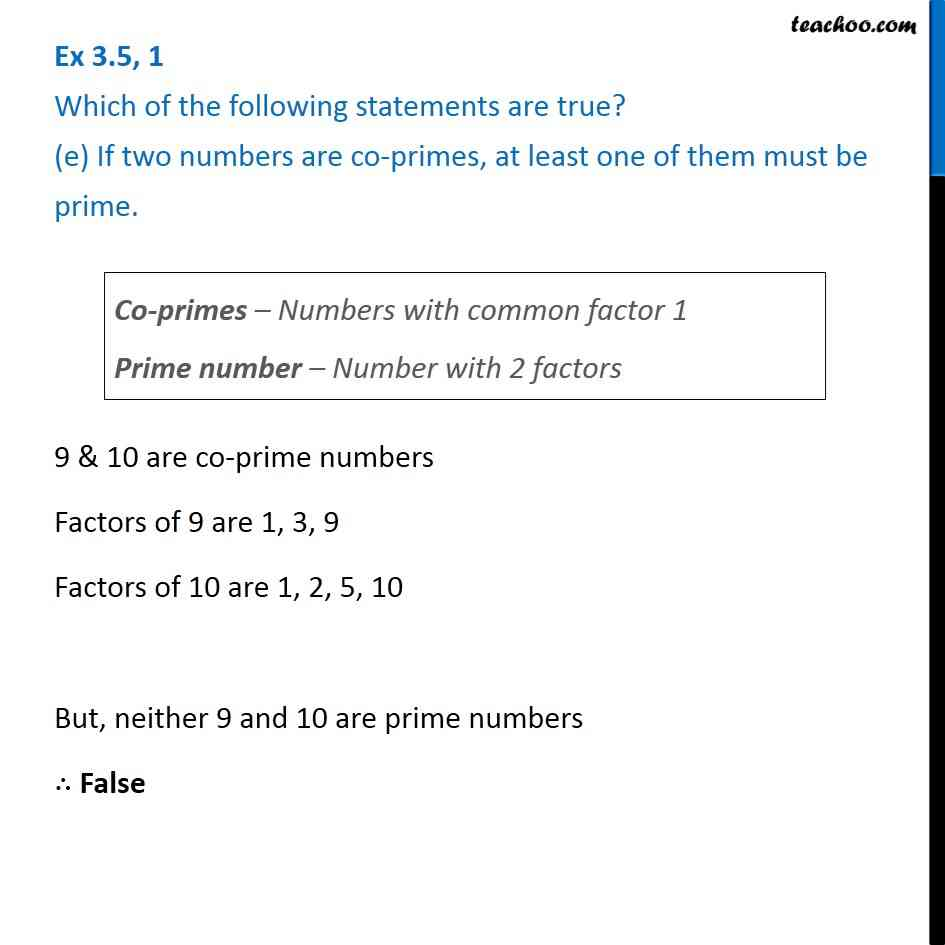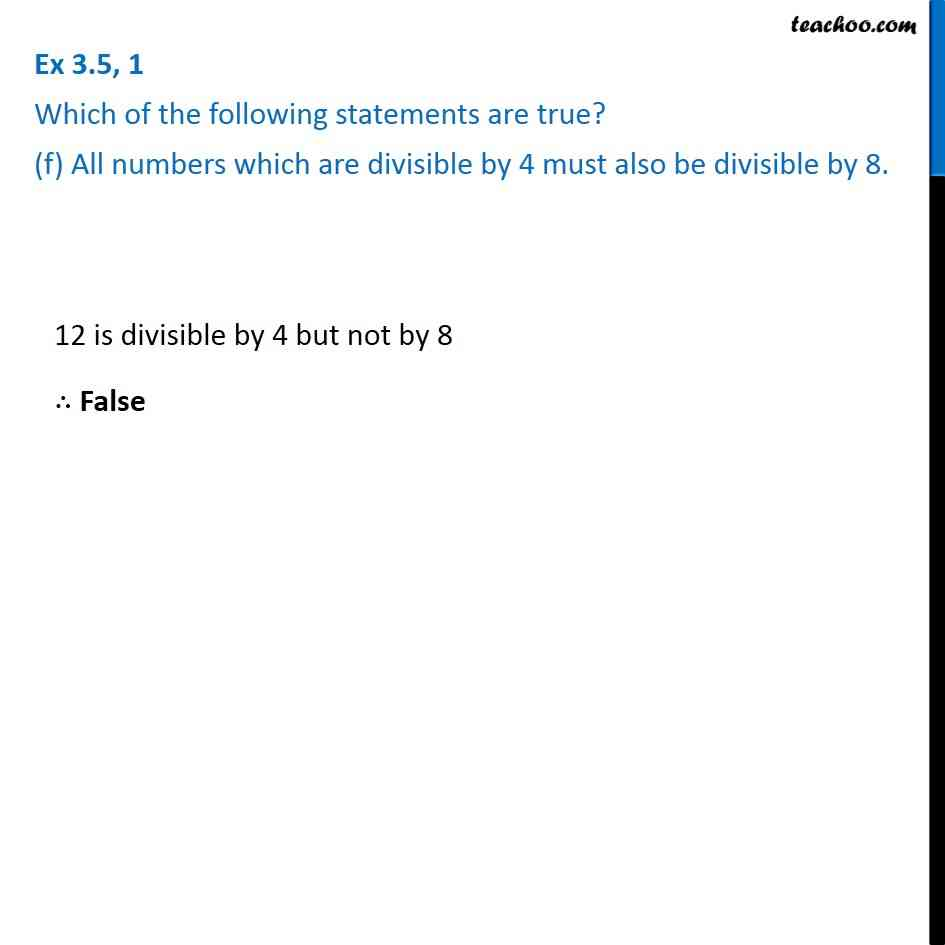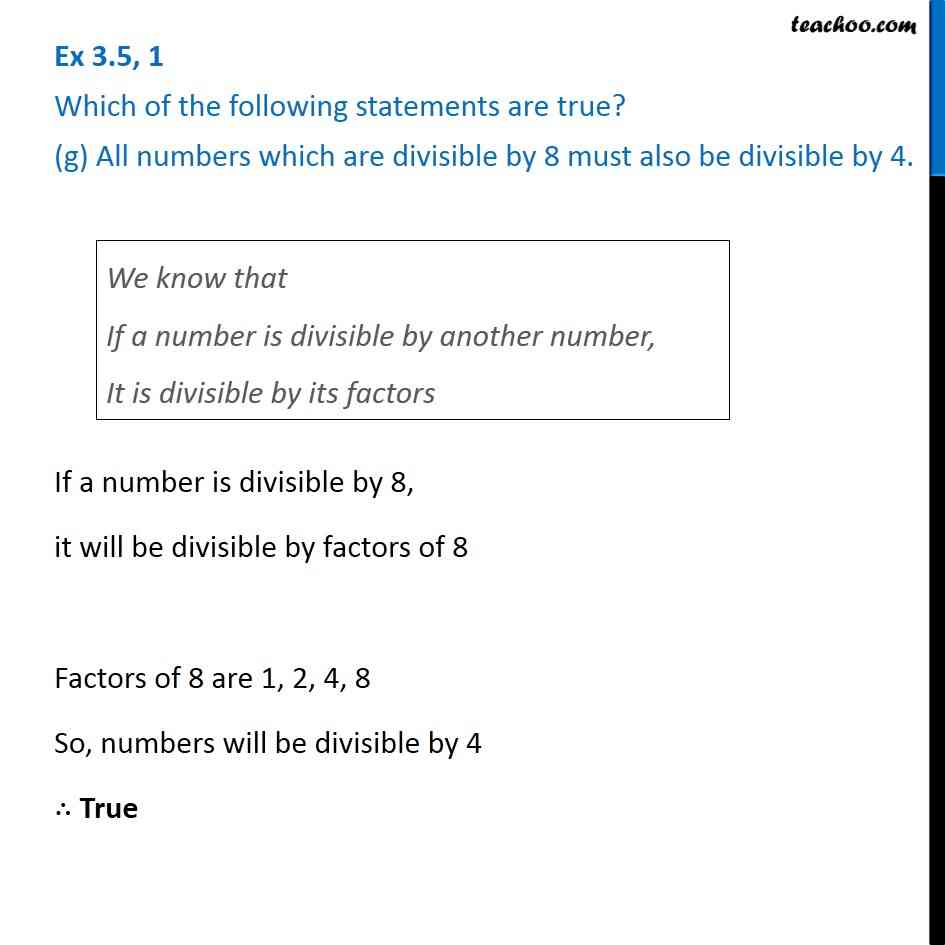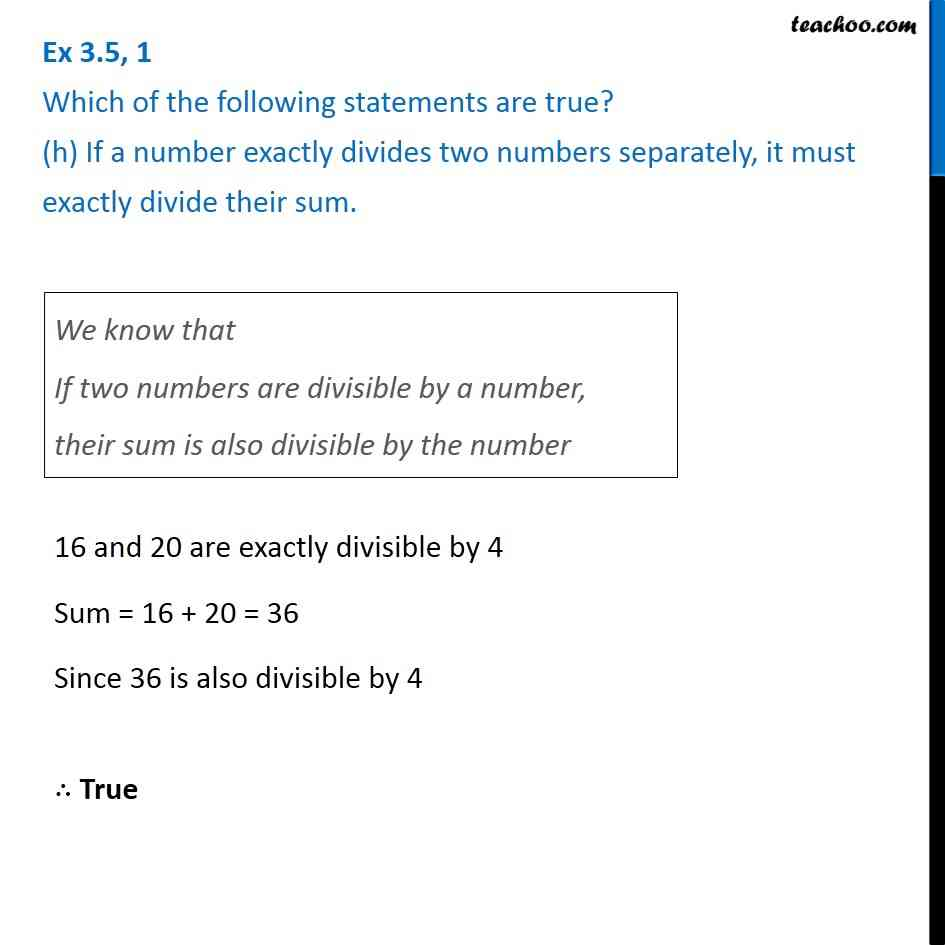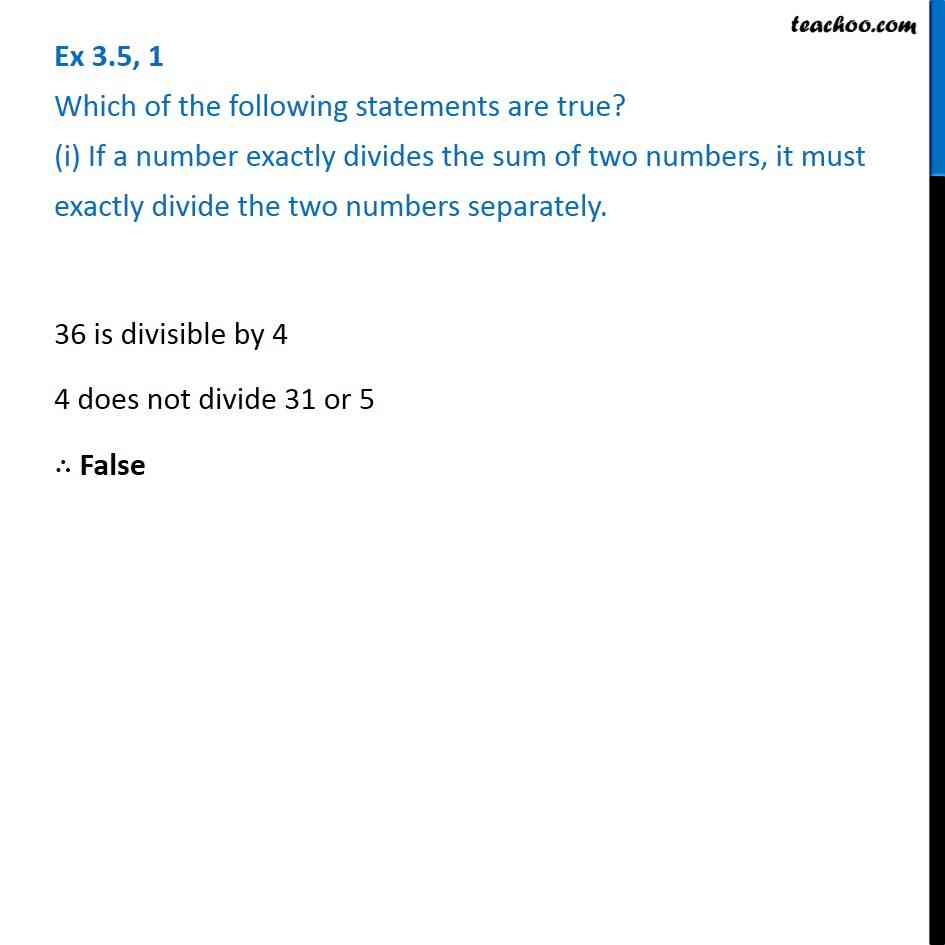Subscribe to our Youtube Channel - https://you.tube/teachoo

1. Chapter 3 Class 6 Playing with Numbers
2. Serial order wise
3. Ex 3.5

Transcript

Ex 3.5, 1 Which of the following statements are true? (a) If a number is divisible by 3, it must be divisible by 9. Ex 3.5, 1 Which of the following statements are true? (b) If a number is divisible by 9, it must be divisible by 3. We know that If a number is divisible by another number, It is divisible by its factors If a number is divisible by 9, it will be divisible by factors of 9 Factors of 9 are 1, 3, 9 So, number will be divisible by 3 ∴ True Ex 3.5, 1 Which of the following statements are true? (c) A number is divisible by 18, if it is divisible by both 3 and 6. We know that If a number is divisible by another number, It is divisible by its factors If a number is divisible by 18, it will be divisible by factors of 18 Factors of 18 are 1, 2, 3, 6, 9, 18 So, number will be divisible by 3 & 6 ∴ True Ex 3.5, 1 Which of the following statements are true? (d) If a number is divisible by 9 and 10 both, then it must be divisible by 90.We know that If a number is divisible by two co-prime numbers, it is divisible their product Checking whether 9 & 10 are co-prime Factors of 9 are 1, 3, 9 Factors of 10 are 1, 2, 5, 10 Since common factor is 1 ∴ 9 & 10 are co-prime So, if a number divisible by 9 and 10 both, then it must be divisible by it product 90 as well ∴ True. Ex 3.5, 1 Which of the following statements are true? (e) If two numbers are co-primes, at least one of them must be prime.Co-primes – Numbers with common factor 1 Prime number – Number with 2 factors 9 & 10 are co-prime numbers Factors of 9 are 1, 3, 9 Factors of 10 are 1, 2, 5, 10 But, neither 9 and 10 are prime numbers ∴ False Ex 3.5, 1 Which of the following statements are true? (f) All numbers which are divisible by 4 must also be divisible by 8. 12 is divisible by 4 but not by 8 ∴ False Ex 3.5, 1 Which of the following statements are true? (g) All numbers which are divisible by 8 must also be divisible by 4.We know that If a number is divisible by another number, It is divisible by its factors If a number is divisible by 8, it will be divisible by factors of 8 Factors of 8 are 1, 2, 4, 8 So, numbers will be divisible by 4 ∴ True Ex 3.5, 1 Which of the following statements are true? (h) If a number exactly divides two numbers separately, it must exactly divide their sum.We know that If two numbers are divisible by a number, their sum is also divisible by the number 16 and 20 are exactly divisible by 4 Sum = 16 + 20 = 36 Since 36 is also divisible by 4 ∴ True Ex 3.5, 1 Which of the following statements are true? (i) If a number exactly divides the sum of two numbers, it must exactly divide the two numbers separately.36 is divisible by 4 4 does not divide 31 or 5 ∴ False

Ex 3.5